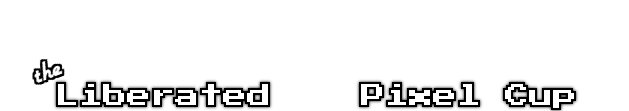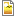## Asteroid Tileset 01

My first "enemy" for the Galaxus game. I've also included the dimensions for each tile because this on is quite obscure. If you're familiar with the XNA C# syntax you don't need this code bit, if you don't the syntax is below. There are three different sizes for each. I've intended for the spritesheet to have this because when you shoot the asteroid it breaks up into different pieces. Each size has eight tiles to it rotating it 45 degrees further. Run through all eight and you get your animation.

Rectangle( "x-position", "y-position", "width", "height");

Level 0:

1. Rectangle(0, 0, 200, 165);
2. Rectangle(200, 0, 187, 183);
3. Rectangle(387, 0, 167, 200);
4. Rectangle(554, 0, 184, 189);
5. Rectangle(738, 0, 200, 165);
6. Rectangle(938, 0, 189, 184);
7. Rectangle(1127, 0, 165, 200);
8. Rectangle(1292, 0, 183, 187);

Level 1:

1. Rectangle(0, 200, 100, 83);
2. Rectangle(100, 200, 93, 92);
3. Rectangle(193, 200, 83, 101);
4. Rectangle(276, 200, 93, 95);
5. Rectangle(369, 200, 101, 83);
6. Rectangle(470, 200, 95, 92);
7. Rectangle(565, 200, 84, 101);
8. Rectangle(649, 200, 92, 95);

Level 2:

1. Rectangle(0, 301, 50, 41));
2. Rectangle(50, 301, 47, 46));
3. Rectangle(97, 301, 41, 51));
4. Rectangle(138, 301, 47, 47));
5. Rectangle(185, 301, 51, 41));
6. Rectangle(236, 301, 47, 46));
7. Rectangle(283, 301, 42, 51));
8. Rectangle(325, 301, 46, 47));Asteroid1.png 442.3 Kb [796 download(s)]